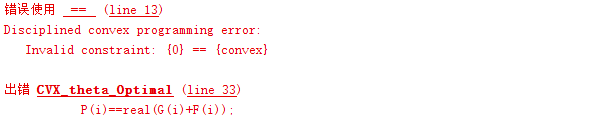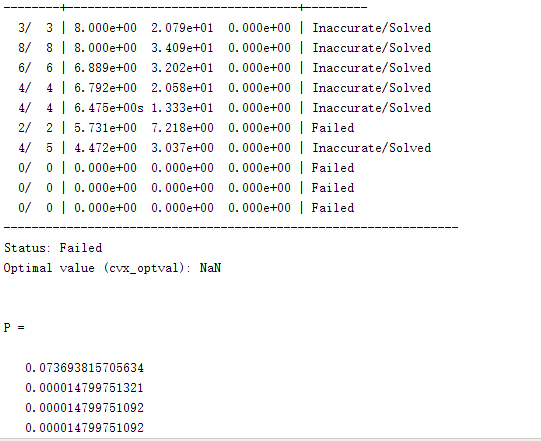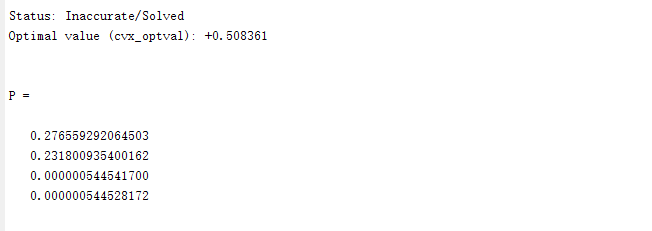# The solution of cvx

P(i) = real(G(i))+F(i) should be P(i) == real(G(i))+F(i). pls read the,cvx users’ guide.This error is like this, P is an expression about Q, it is not a number.

function [Q_,P_] = CVX_theta_Optimal(M,P_max,K,Z,v,q,p,BER)

cvx_begin
cvx_quiet false;
variable Q(M+1,M+1) hermitian semidefinite
variable P(K)

``````expression F(K)
expression G(K)
expression A(K)

for i=1:K
if i == 1
s_inter = 0;
G_tmp = 0;
else
for j=1:i-1
s_inter = p(j).*(trace(Z(:,:,j)*q)+ norm((v(:,j)))) + s_inter;
G_tmp = G_tmp + Z(:,:,j)';
end
end
F(i) = -log(real(trace(Z(:,:,i)*Q)+norm(v(:,i))))/log(2);
end
``````

% expression N
%
%
minimize sum§
subject to
for i=1:K
0<P(i)<=P_max;
end
diag(Q) == 1 ;
for i = 1:K
A(i) = 2.^((real(real(G(i))+F(i))+log(power((5.5+sqrt(1/200)*qfuncinv(BER)/log(2)),2)-1)/log(2)));
A(i)<=P(i);
end
% N = 0;

cvx_end
I change my code to this ,but this solution is very small,it not satisfy this constraint.function [Q_,P_] = CVX_theta_Optimal(M,P_max,K,Z,v,q,p,BER)

cvx_begin
cvx_quiet false;
variable Q(M+1,M+1) hermitian semidefinite
variable P(K)
expression F(K)
expression G(K)
for i=1:K
if i == 1
s_inter = 0;
G_tmp = 0;
else
for j=1:i-1
s_inter = p(j).(trace(Z(:,:,j)q)+ norm((v(:,j)))) + s_inter;
G_tmp = G_tmp + Z(:,:,j)’;
end
end
(s_inter+1);
G(i) = -rel_entr(1,real(s_inter+1))+2
F(i) = -(-rel_entr(1,(real(trace(Z(:,:,i)Q)+norm(v(:,i))))))/log(2);
end
% expression N
% N = norm_nuc(Q)-norm(q)+2
minimize P(1)+P(2)+P(3)+P(4)
subject to
for i=1:K
0<=P(i)<=P_max;
end
diag(Q) == 1 ;
for i = 1:K
P(i)-exp(log(2)
(log(2.^(2+(sqrt(1/200)*qfuncinv(BER)/log(2)))-1)/log(2)+real(real(G(i))+F(i))))>=0;
end
% N = 0;

cvx_end
%Q
P
Q_ = Q;
P_ = P;

endI change my code to this.Although the optimal value can be generated, it is still not satisfied with the constraint, and the gap is very large. Sometimes NAN also occur.Can you help me see what is the problem?

Thank you !Hope you can help me take a look at this problem.

You haven’t shown us the output. We don’t even know what solution method was used. Note that constrains are not satisfied exactly, but only to within a tolerance. And CVX reported that your problem was solved inaccurately. It is possible that your input data is not well-scaled.

Sorry，This is my code and output.
function [Q_,P_] = CVX_theta_Optimal(M,P_max,K,Z,v,q,p,BER)

cvx_solver mosek
cvx_begin
cvx_quiet false;
variable Q(M+1,M+1) hermitian semidefinite

``````expression F(K)
expression G(K)
expression P(K)

for i=1:K
if i == 1
s_inter = 0;
G_tmp = 0;
else
s_inter = 0;
G_tmp = 0;
for j=1:i-1
s_inter = p(j).*(trace(Z(:,:,j)*q)+ sum(abs(v(:,i)).^2)) + s_inter;
G_tmp = G_tmp + Z(:,:,j).';
end
end
F(i) = -(-rel_entr(1,(real(trace(Z(:,:,i)*Q)+sum(abs(v(:,i)).^2)))))/log(2);
end
%expression N
minimize sum(P)
subject to
diag(Q) == 1 ;

for i=1:K
0<=P(i)<=P_max;
end

for i=1:K
P(i)-exp(log(2)*(log(2.^(1+(sqrt(1/200)*qfuncinv(BER)/log(2)))-1)/log(2)+real(G(i)+F(i))))>=0;
end

%N = 0;
``````

cvx_end
%Q
P
Q_ = Q;
P_ = P;

## Cones | Errors | Mov/Act | Centering Exp cone Poly cone | Status --------±--------------------------------±-------- 0/ 2 | 8.000e+00 6.868e+02 3.299e+43 | Infeasible

Status: Infeasible
Optimal value (cvx_optval): +Inf

P =

NaN
NaN
NaN
NaN

I don’t know why this problem occurs. I don’t know how to modify my code to make it run normally. Please help me, thank you!!!

Sorry，This is my code and output.

if the problem is still reported infeasible, follow the advice (except for section 1) at https://yalmip.github.io/debugginginfeasible .

Thank you very much！

Actually, as I wrote in the other thread, make sure you are using CVX 2.2 and Mosek 9.x. Otherwise you would not be getting the output with Cones | Errors … which shows the Successive approximation method was used.

OK，Thank you！I change my CVX version.

I have another question, when should I use cvx_quad?

As long as your problem can reformulated using the exponential cone and symmetric cones you should not use it.

Thank you very much!

I have another question that needs your help

When I solving the SOCP problem, the output is Nan?
this my problem

This is my code and output.
%% 参数
clc;clear ;
Z_mc=1;
c=3e8;
f =28e9;%f=18.5e+9; 8e-3=0.008 0.5lambda
lambda=c/f;
dx=5e-3;% 0.5lambda
dy=dx;
Ny=8;Nx=8;
kc=1j *2 *pi /lambda;
theta_0=0;%目标角度 *pi/180
phi_0=0;
% theta_i=30;%干扰角度 *pi/180
% phi_i=0;
% INR=30;
u=10.^(1./10);%主瓣阈值（上限）
l=10.^(-1./10);%主瓣阈值（下限）
taos=0.001;%旁瓣阈值（上限）30dB taos=0.001;%旁瓣阈值（上限）=10.^(-30./10); -30db
%% 目标导向矢量
a0_x=exp(kc *(0:Nx-1)’ *dx *sin(theta_0 *pi/180) *cos(phi_0 *pi/180));
a0_y=exp(kc *(0:Ny-1)’ *dy *sin(theta_0 *pi/180) *sin(phi_0 pi/180));
a0=kron(a0_x, a0_y);%导向矢量
a=a0’ Z_mc;%耦合导向矢量 1N
%% 主瓣导向矢量
theta_m=(-10:10);
phi_m=(-10:10);
M =length(theta_m);
for m=1:M
am_x=exp(kc * (0:Nx-1)’ * dx * sin(theta_m(m) * pi/180) * cos(phi_m(m) * pi/180));
am_y=exp(kc * (0:Ny-1)’ * dy * sin(theta_m(m) * pi/180) * sin(phi_m(m) * pi/180));
a10=kron(am_x, am_y);%导向矢量
a1(m,:)=a10’ * Z_mc;%耦合导向矢量 1N
end
%% 旁瓣导向矢量
theta_s=[-91:4:-9,11:4:91];
phi_s=[-91:4:-9,11:4:91];
S =length(theta_s);
for s=1:S
as_x=exp(kc * (0:Nx-1)’ * dx * sin(theta_s(s) * pi/180) * cos(phi_s(s) pi/180));
as_y=exp(kc * (0:Ny-1)’ * dy * sin(theta_s(s) * pi/180) * sin(phi_s(s) * pi/180));
a20=kron(as_x, as_y);%导向矢量
a2(s,:)=a20’ * Z_mc;%耦合导向矢量 1N
end
N=Ny * Nx;
%% cvx
yita=(u-l) * sqrt(Ny * Nx)/2;%导向矢量误差
cvx_begin
cvx_solver SeDuMi
variable w(N) complex %N1
minimize(norm(w))%优化目标
subject to
a * w==1;
for m=1:1:M
real(a1(m, : ) * w) -yita * norm(w) >= l;%
norm(a1(m, : ) * w)+yita * norm(w) <= u;%
end
for s=1:1:S
norm(a2(s,: ) * w) +yita * norm(w)<= taos;%
end
cvx_end
% wopt=w% N1